## Gauge Symmetry in Quantum Mechanics

Gauge symmetry in Electromagnetism was recognized before the advent of quantum mechanics. We have seen that symmetries play a very important role in the quantum theory. Indeed, in quantum mechanics, gauge symmetry can be seen as the basis for electromagnetism and conservation of charge.

We know that the all observables are unchanged if we make a global change of the phase of the wavefunction,. We could call this global phase symmetry.All relative phases (say for amplitudes to go through different slits in a diffraction experiment) remain the same and no physical observable changes. This is a symmetry in the theory which we already know about. Let's postulate that there is a bigger symmetry and see what the consequences are.That is, we can change the phase by a different amount at each point in spacetime and the physics will remain unchanged. This local phase symmetry is bigger than the global one.

Its clear that this transformation leaves the absolute square of the wavefunction the same, but what about the Schrödinger equation? It must also be unchanged. The derivatives in the Schrödinger equation will act onchanging the equation unless we do something else to cancel the changes.A little calculation shows that the equation remains unchanged if we also transform the potentials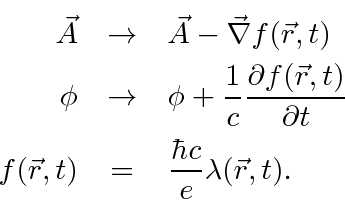This is just the standard gauge transformation of electromagnetism, but, we now see that local phase symmetry of the wavefunction requires gauge symmetry for the fields and indeed even requires the existence of the EM fields to cancel terms in the Schrödinger equation. Electromagnetism is called a gauge theory because the gauge symmetry actually defines the theory. It turns out that the weak and the strong interactions are also gauge theories and, in some sense, have the next simplest possible gauge symmetries after the one in Electromagnetism.

We will write our standard gauge transformation in the traditional way to conform a bit better to the textbooks.There are measurable quantum physics consequences of this symmetry. We can understand a number of them by looking at the vector potential in a field free regions. Ifthencan be written as the gradient of a function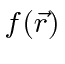. To be specific, take our gauge transformation of the vector potential. Make a gauge transformation such that. This of course is still consistent with.Then the old vector potential is then given by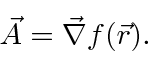Integrating this equation, we can write the functionin terms of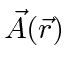.If we chooseso that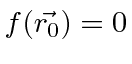, then we have a very useful relation between the gauge function and the vector potential in a field free region.We can derive the quantization of magnetic flux by calculating the line integral of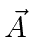around a closed loop in a field free region.A good example of aregion is a superconductor. Magnetic flux is excluded from the superconducting region. If we have a superconducting ring, we have a B=0 region surrounding some flux. We have shown then, that the flux going through a ring of superconductor is quantized.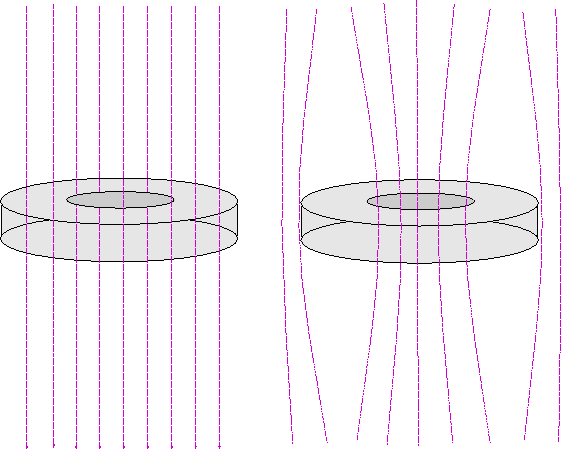Flux is observed to be quantized but the charge of the particle seen is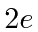.This is due to the pairing of electrons inside a superconductor.

The Aharanov Böhm Effect brings us back to the two slit diffraction experiment but adds magnetic fields.The electron beams travel through two slits in field free regions but we have the ability to vary a magnetic field enclosed by the path of the electrons. At the screen, the amplitudes from the two slits interfere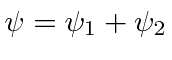. Let's start withandeverywhere. When we change thefield, the wavefunctions must change.The relative phase from the two slits depends on the flux between the slits. By varying thefield, we will shift the diffraction pattern even thoughalong the whole path of the electrons. While this may at first seem amazing, we have seen similar effects in classical E&M with an EMF induced in a loop by a changingfield which does not touch the actual loop.

Jim Branson 2013-04-22